Digital Logic | Full Subtractor

A full subtractor is a combinational circuit that performs subtraction of two bits, one is minuend and other is subtrahend, taking into account borrow of the previous adjacent lower minuend bit. This circuit has three inputs and two outputs. The three inputs A, B and Bin, denote the minuend, subtrahend, and previous borrow, respectively. The two outputs, D and Bout represent the difference and output borrow, respectively.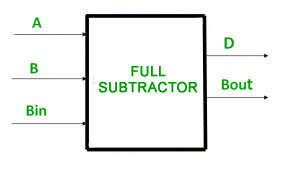Truth Table –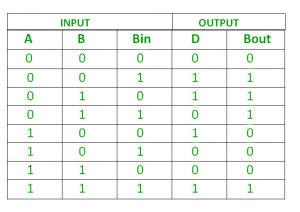From above table we can draw the K-Map as shown for “difference” and “borrow”.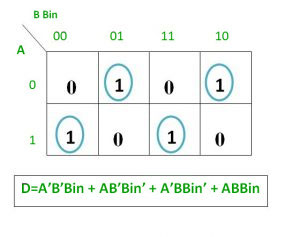Logical expression for difference –

D   = A’B’Bin + A’BBin’ + AB’Bin’ + ABBin
= Bin(A’B’ + AB)  + Bin’(AB’ + A’B)
= Bin( A XNOR B) + Bin’(A XOR B)
= Bin (A XOR B)’  +  Bin’(A XOR B)
= Bin XOR (A XOR B)
= (A XOR B) XOR Bin

Logical expression for borrow –

Bout = A’B’Bin + A’BBin’ + A’BBin + ABBin
= A’B’Bin +A’BBin’ + A’BBin + A’BBin + A’BBin + ABBin
= A’Bin(B + B’) + A’B(Bin + Bin’) + BBin(A + A’)
= A’Bin + A’B + BBin

OR

Bout = A’B’Bin + A’BBin’ + A’BBin + ABBin
= Bin(AB + A’B’) + A’B(Bin + Bin’)
= Bin( A XNOR B) + A’B
= Bin (A XOR B)’ + A’B

Logic Circuit for Full Subtractor –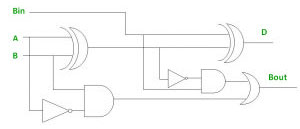Implementation of Full Subtractor using Half Subtractors –
2 Half Subtractors and an OR gate is required to implement a Full Subtractor.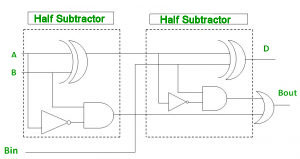Reference – Full Subtractor – Wikipedia

This article is contributed by Harshita Pandey. If you like GeeksforGeeks and would like to contribute, you can also write an article using contribute.geeksforgeeks.org or mail your article to contribute@geeksforgeeks.org. See your article appearing on the GeeksforGeeks main page and help other Geeks.

Please write comments if you find anything incorrect, or you want to share more information about the topic discussed above.My Personal Notes arrow_drop_up